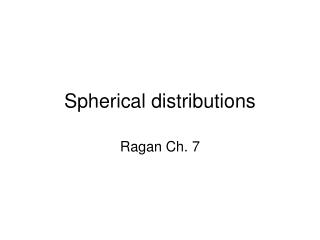DownloadDownload PresentationSpherical distributions

# Spherical distributions

Télécharger la présentation## Spherical distributions

- - - - - - - - - - - - - - - - - - - - - - - - - - - E N D - - - - - - - - - - - - - - - - - - - - - - - - - - -
##### Presentation Transcript

1. Spherical distributions Ragan Ch. 7

2. Analyze a cluster of points on a sphere. How do you determine the mean orientation and how clustered the data are? Mean direction is given by resultant vector

3. mean plunge = 40.0 mean trend = 208.6 R_bar is 0.96 [L, M, N] =plunge_trend_to_dir_cosines(p,t); Rx = sum(L); Ry = sum(M); Rz = sum(N); R_mag = sqrt(Rx.^2 + Ry.^2 + Rz.^2); l_bar = Rx./R_mag; m_bar = Ry./R_mag; n_bar = Rz./R_mag; [plunge, trend] = dir_cosines_to_plunge_trend(l_bar, m_bar, n_bar);

4. Ramon South Mountains joints

5. Ramon South Mountains joints mean plunge = 38.3 mean trend = 216.7 and R_bar is 0.45

6. Ramon South Mountains joints cleaned mean plunge = 26.9 mean trend = 216.7 and R_bar is 0.62